# Logistic Regression in Machine Learning

In this page we will learn What is Logistic Regression in Machine Learning?, Logistic Function (Sigmoid Function), Logistic Regression Equation, Type of Logistic Regression, Python Implementation of Logistic Regression (Binomial), Type of Logistic Regression, Data Pre-processing step, Fitting Logistic Regression to the Training set, Linear Classifier.

## What is Logistic Regression in Machine Learning?

Under the Supervised Learning approach, one of the most prominent Machine Learning algorithms is logistic regression. It's a method for predicting a categorical dependent variable from a set of independent variables.
A categorical dependent variable's output is predicted using logistic regression. As a result, the result must be a discrete or categorical value. It can be Yes or No, 0 or 1, true or false, and so on, but instead of giving exact values like 0 and 1, it delivers probabilistic values that are somewhere between 0 and 1.
Except for how they are employed, Logistic Regression is very similar to Linear Regression. For regression problems, Linear Regression is employed, while for classification difficulties, Logistic Regression is used. Instead of fitting a regression line, we fit a "S" shaped logistic function in logistic regression, which predicts two maximum values (0 or 1).
The logistic function's curve reflects the probability of things like whether the cells are cancerous or not, whether a mouse is obese or not based on its weight, and so on.
Because it can generate probabilities and classify new data using both continuous and discrete datasets, logistic regression is a key machine learning approach.
Logistic regression may be used to categorize observations based on many forms of data and can quickly identify the most useful factors for classification. The logistic function is depicted in the graphic below: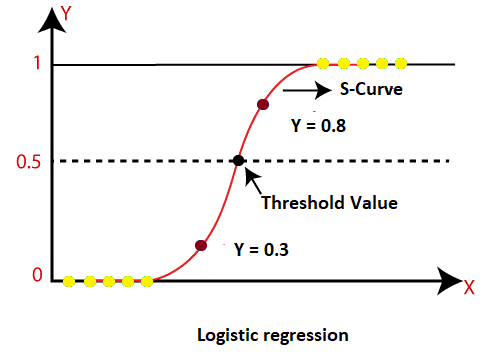Note: Logistic regression is a classification algorithm that leverages the concept of predictive modeling as regression. It is termed logistic regression because it is used to categorize data.

## Logistic Function (Sigmoid Function):

• The sigmoid function is a mathematical formula for converting anticipated values into probabilities.
• It converts any real value between 0 and 1 into another value.
• The logistic regression's value must be between 0 and 1, and it cannot exceed this limit, resulting in a "S" curve. The Sigmoid function, often known as the logistic function, is the S-form curve.
• The concept of the threshold value is used in logistic regression to describe the probability of either 0 or 1. For example, numbers above the threshold value likely to be 1, whereas values below the threshold value tend to be 0.

Assumptions for Logistic Regression:

• The dependent variable needs to be categorical.
• There should be no multi-collinearity in the independent variable.

## Logistic Regression Equation:

The Linear Regression Equation can be used to get the Logistic Regression Equation. The following are the mathematical steps to obtain Logistic Regression equations:

• We know that the straight line equation can be represented as:

### y = b0 + b1x1 + b2x2 + ..... + bnxn

• Because y in Logistic Regression may only be between 0 and 1, divide the previous equation by (1-y):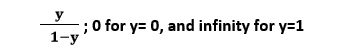• However, we require a range of -[infinity] to +[infinity], in which case the logarithm of the equation is: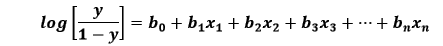The final equation for Logistic Regression is as follows.

## Type of Logistic Regression:

Logistic regression can be divided into three types based on the categories:

• Binomial: In binomial Logistic regression, the dependent variables can only be one of two types, such as 0 or 1, Pass or Fail, and so on.
• Multinomial: In multinomial logistic regression, the dependent variable might be one of three or more unordered kinds, such as ""cats," "dogs," or "sheep" are all examples of animal names.
• Ordinal: This Logistic Regression allows for three or more ordered sorts of dependent variables, such as "low," "medium," or "high."

## Python Implementation of Logistic Regression (Binomial)

To understand the implementation of Logistic Regression in Python, we will use the below example:

For example, a dataset is provided that contains information from various people acquired from social networking sites. There is a car manufacturer that has lately released a new SUV vehicle. As a result, the corporation sought to see how many consumers in the dataset desired to buy the car.

We'll use the Logistic Regression approach to create a Machine Learning model for this problem. The dataset is depicted in the graphic below. We will use age and salary to forecast the purchased variable (Dependent Variable) in this problem (Independent variables).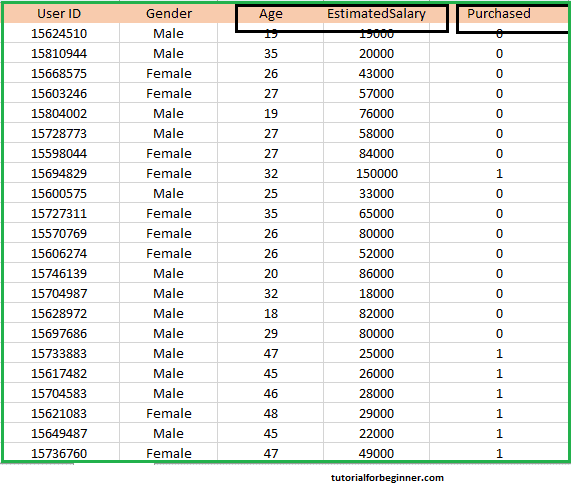## Type of Logistic Regression:

We'll utilize the same steps we used in earlier Regression topics to implement Logistic Regression in Python. The steps are as follows:

• Data Pre-processing step
• Fitting Logistic Regression to the Training set
• Predicting the test result
• Test accuracy of the result(Creation of Confusion matrix)
• Visualizing the test set result.

## 1. Data Pre-processing step:

In this stage, we will pre-process/prepare the data so that we may use it effectively in our code. It will be similar to what we did in the Data Pre-processing topic. The following is the code for this:

``````
#Data Pre-procesing Step
#importing libraries
import numpy as nm
import matplotlib.pyplot as mtp
import pandas as pd
#importing datasets
```
```

We will get the dataset as an output by running the aforementioned lines of code. Consider the following illustration: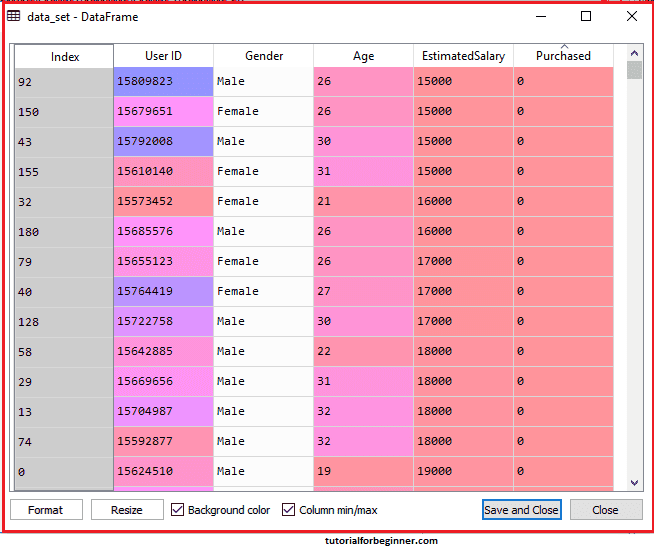From the above dataset, we will now extract the dependent and independent variables. The code for it is as follows:

``````
#Extracting Independent and dependent Variable
x = data_set.iloc[:, [2,3]].values
y = data_set.iloc[:, 4].values
```
```

Because our independent variables, age and salary, are at index 2, 3, we used [2, 3] for x in the above code. We chose 4 for the y variable because our dependent variable is indexed at 4. The end result will be: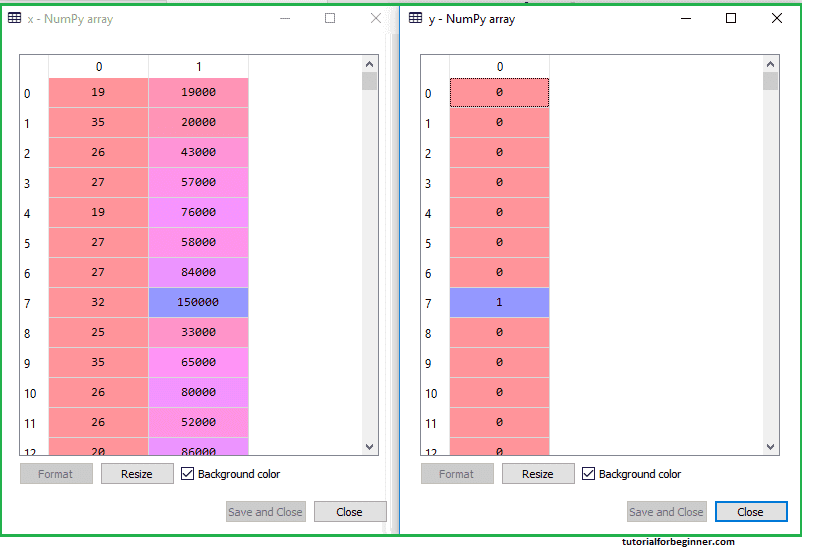Now we will split the dataset into a training set and test set. Below is the code for it:

``````
#Splitting the dataset into training and test set.
from sklearn.model_selection import train_test_split
x_train, x_test, y_train, y_test = train_test_split(x, y, test_size = 0.25, random_state = 0)
```
```

The output for this is given below: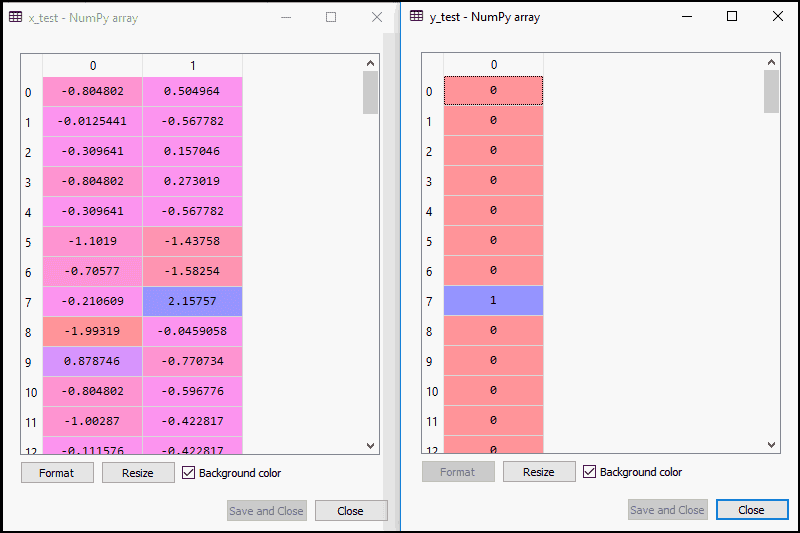## For training set: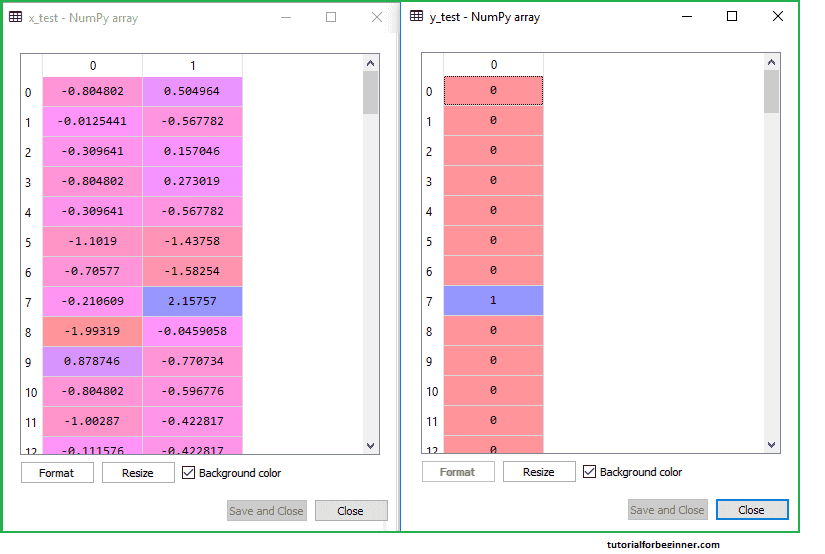We will utilize feature scaling in logistic regression since we want precise predictions. Because the dependent variable only has 0 and 1 values, we shall only scale the independent variable. The code for it is as follows:

``````
#feature Scaling
from sklearn.preprocessing import StandardScaler
st_x = StandardScaler()
x_train = st_x.fit_transform(x_train)
x_test = st_x.transform(x_test)
```
```

The scaled output is given below: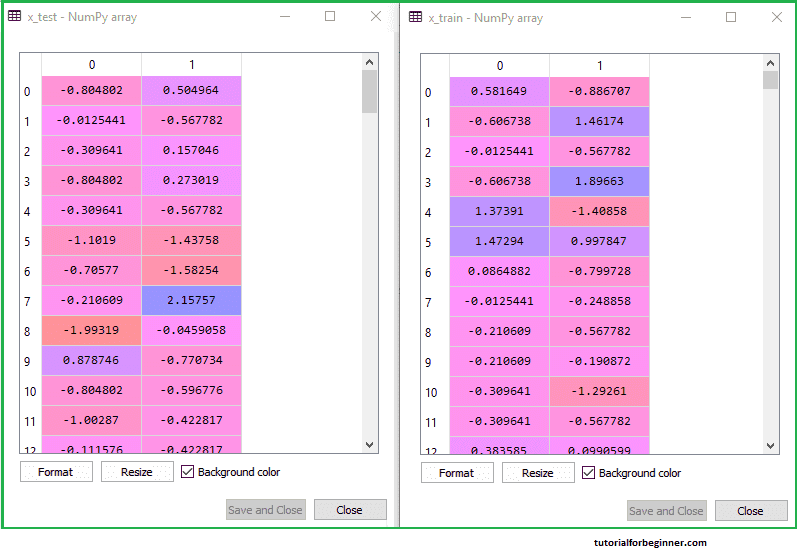## 2. Fitting Logistic Regression to the Training set:

We've carefully prepared our dataset, and now we'll use the training set to train it. We'll use the sklearn library's LogisticRegression class to provide training or fit the model to the training set.
We'll create a classifier object after importing the class and use it to fit the model to the logistic regression. The code for it is as follows:

``````
#Fitting Logistic Regression to the training set
from sklearn.linear_model import LogisticRegression
classifier = LogisticRegression(random_state = 0)
classifier.fit(x_train, y_train)
```
```

## Output:

By executing the above code, we will get the below output:

## Out:

``````
LogisticRegression( C = 1.0, class_weight = None, dual = False, fit_intercept = True,
intercept_scaling = 1, l1_ratio = None, max_iter = 100, multi_class = 'warn',
n_jobs = None, penalty = 'l2', random_state = 0, solver = 'warn', tol = 0.0001,
verbose = 0, warm_start = False )
```
```

Therefore, our model is well fitted to the training set.

## 3. Predicting the Test Result

Because our model has been well-trained on the training set, we will now use test set data to predict the outcome. The code for it is as follows:

``````
#Predicting the test set result
y_pred = classifier.predict(x_test)
In the above code, we have created a y_pred vector to predict
the test set result.
```
```

## Output:

When the preceding code is run, a new vector (y pred) is created in the variable explorer option. It can be summed up as follows: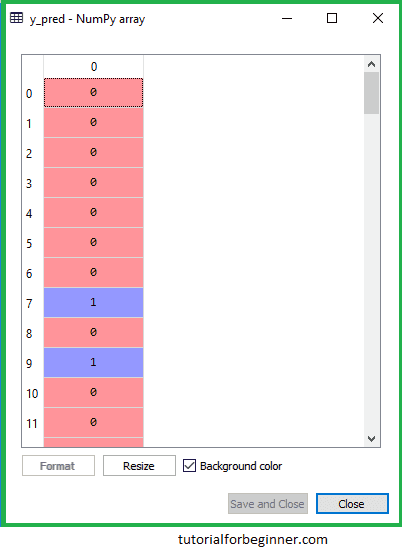The expected users who desire to buy or not buy the car are depicted in the above output image.

## 4. Test Accuracy of the result

Now we'll make a confusion matrix to see how accurate the classification is. To make it, we'll need to import the sklearn library's confusion matrix function. We'll use a new variable cm to call the function when it's been imported. The function has two parameters: y true (the actual values) and y pred (the predicted values) (the targeted value return by the classifier). The code for it is as follows:

``````
#Creating the Confusion matrix
from sklearn.metrics import confusion_matrix
cm = confusion_matrix()
```
```

## Output:

By executing the above code, a new confusion matrix will be created. Consider the below image: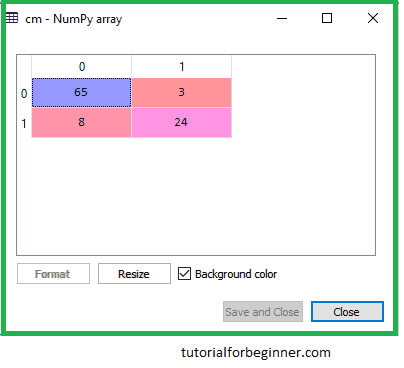The expected users who desire to buy or not buy the car are depicted in the above output image.

## 5. Visualizing the training set result

Finally, we'll display the results of the training set. We'll use the matplotlib library's ListedColormap class to show the output. The code for it is as follows:

``````
#Visualizing the training set result
from matplotlib.colors import ListedColormap

x_set, y_set = x_train, y_train
x1, x2 = nm.meshgrid(nm.arange(start = x_set[:, 0].min() - 1,
stop = x_set[:, 0].max() + 1, step = 0.01),
nm.arange(start = x_set[:, 1].min() - 1, stop = x_set[:,
1].max() + 1, step = 0.01))
mtp.contourf(x1, x2, classifier.predict(nm.array([x1.ravel(),
x2.ravel()]).T).reshape(x1.shape),
alpha = 0.75, cmap = ListedColormap(('purple','green' )))
mtp.xlim(x1.min(), x1.max())
mtp.ylim(x2.min(), x2.max())

for i, j in enumerate(nm.unique(y_set)):
mtp.scatter(x_set[y_set == j, 0], x_set[y_set == j, 1],
c = ListedColormap(('purple', 'green'))(i), label = j)
mtp.title('Logistic Regression (Training set)')
mtp.xlabel('Age')
mtp.ylabel('Estimated Salary')
mtp.legend()
mtp.show()
```
```

We used the Matplotlib library's ListedColormap class to construct the colormap for viewing the outcome in the above code. To replace x_train and y_train, we've established two new variables, x_set and y_set. Following that, we used the nm.meshgrid command to generate a rectangular grid with a range of -1 (minimum) to 1 (maximum) (maximum). The pixel points we took had a resolution of 0.01. We used the mtp.contourf command to build a filled contour; it will construct areas of the supplied colors (purple and green). We gave the classifier.predict parameter to this function to display the classifier's predicted data points.

Output: We will get the following result if we run the above code.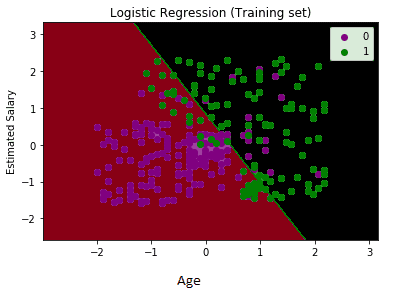The following points can be used to explain the graph:

We can observe some Green points within the green region and Purple points within the purple region in the graph above. All of these data points are observation points from the training set, and they represent the outcome for purchased variables.
The age on the x-axis and the estimated pay on the y-axis are the two independent variables in this graph. The purple point observations are for users who did not acquire the SUV automobile (dependent variable), i.e., users who did not buy the SUV car.
The green point observations are for which vehicle was purchased (dependent variable), which is most likely 1 for the person who bought the SUV car.
We can also deduce from the graph that younger users with lower salaries did not buy the car, whereas older users with higher projected salaries did.
However, some purple points can be found in the green region (vehicle purchase) and some green points can be found in the purple region (Not buying the car). As a result, we can conclude that younger users with a high estimated wage bought the car, but older users with a low estimated salary did not.

## The goal of the classifier:

The training set result for the logistic regression has been successfully visualized, and our purpose for this classification is to divide the users who purchased the SUV automobile from those who did not. As a result, the two zones (Purple and Green) with the observation points are clearly visible in the output graph. Users who did not purchase the car are in the Purple region, while those who did purchase the car are in the Green region.

## Linear Classifier:

The classifier is a straight line or linear in nature, as we utilized the Linear model for Logistic Regression, as we can see from the graph. We shall learn about non-linear Classifiers in future topics.

## Visualizing the test set result:

Using the training dataset, we have a well-trained model. Now we'll see how the result looks for new observations (Test set). The code for the test set will be the same as before, except that instead of x train and y train, we will use x test and y test. The code for it is as follows:

``````
#Visulaizing the test set result
from matplotlib.colors import ListedColormap
x_set, y_set = x_test, y_test
x1, x2 = nm.meshgrid(nm.arange(start = x_set[:, 0].min() - 1, stop = x_set[:, 0].max() + 1, step  = 0.01),
nm.arange(start = x_set[:, 1].min() - 1, stop = x_set[:, 1].max() + 1, step = 0.01))
mtp.contourf(x1, x2, classifier.predict(nm.array([x1.ravel(), x2.ravel()]).T).reshape(x1.shape),
alpha = 0.75, cmap = ListedColormap(('purple','green' )))
mtp.xlim(x1.min(), x1.max())
mtp.ylim(x2.min(), x2.max())
for i, j in enumerate(nm.unique(y_set)):
mtp.scatter(x_set[y_set == j, 0], x_set[y_set == j, 1],
c = ListedColormap(('purple', 'green'))(i), label = j)
mtp.title('Logistic Regression (Test set)')
mtp.xlabel('Age')
mtp.ylabel('Estimated Salary')
mtp.legend()
mtp.show()
```
```

## Output: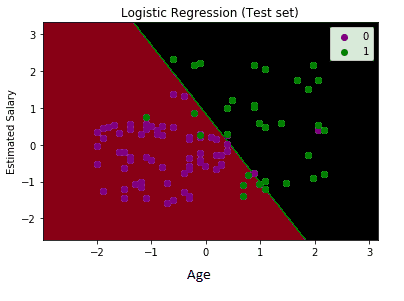The result of the test set is shown in the graph above. The graph is separated into two sections, as can be seen (Purple and Green). Observations in the green region are in the green region, while those in the purple zone are in the purple region. As a result, we can conclude that the forecast and model are accurate. Because we have already estimated this mistake using the confusion matrix, some of the green and purple data points are in different locations, which can be ignored (11 Incorrect output).

As a result, our model is fairly accurate and capable of making new predictions for this categorization challenge.